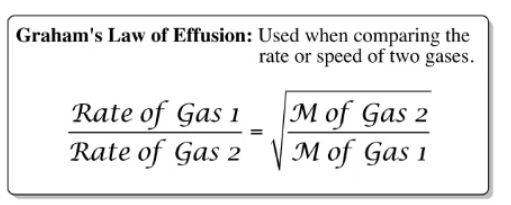# Problem: A gas of unknown molecular mass was allowed to effuse through a small opening under constant-pressure conditions. It required 115 s for 1.0 L of the gas to effuse. Under identical experimental conditions it required 31 s for 1.0 L of O2 gas to effuse.Calculate the molar mass of the unknown gas. (Remember that the faster the rate of effusion, the shorter the time required for effusion of 1.0 L; that is, rate and time are inversely proportional.)

###### FREE Expert Solution

We use Graham's Law of Effusion to solve this problem:Where M is the Molar mass.

We do not have the given rates so we would have to modify the equation to fit the given values.

Now the rate is si given as below:

92% (52 ratings)###### Problem Details

A gas of unknown molecular mass was allowed to effuse through a small opening under constant-pressure conditions. It required 115 s for 1.0 L of the gas to effuse. Under identical experimental conditions it required 31 s for 1.0 L of O2 gas to effuse.

Calculate the molar mass of the unknown gas. (Remember that the faster the rate of effusion, the shorter the time required for effusion of 1.0 L; that is, rate and time are inversely proportional.)